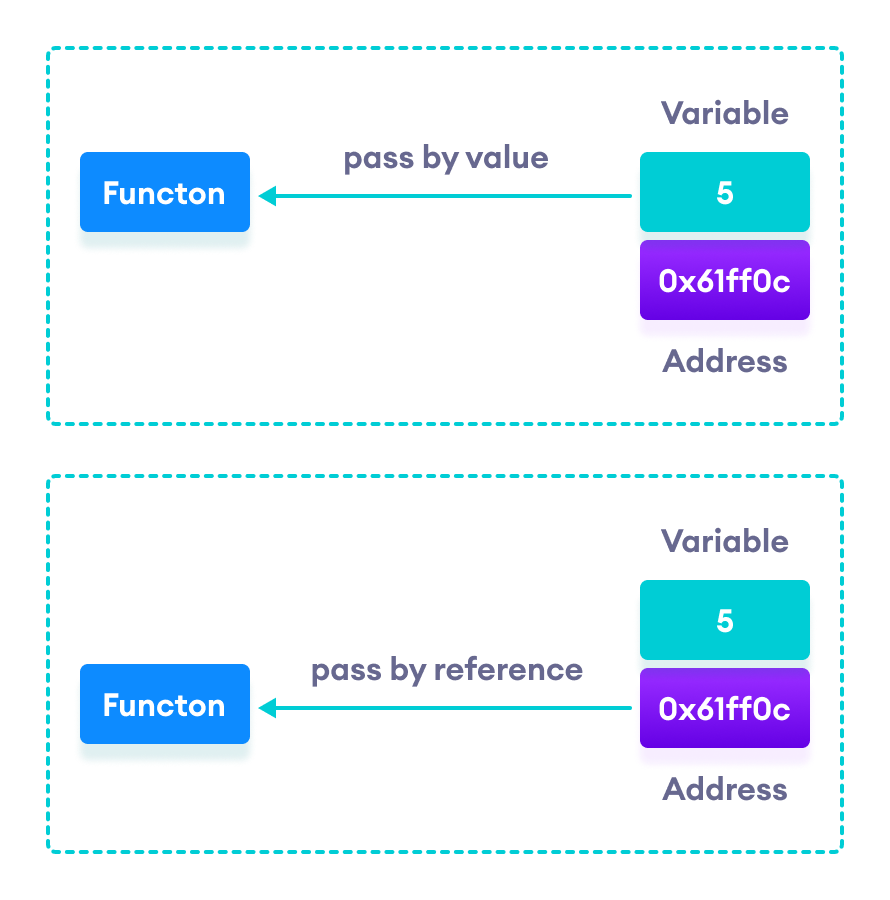# C++ Call by Reference: Using pointers [With Examples]

In this tutorial, we will learn about C++ call by reference to pass pointers as an argument to the function with the help of examples.

In the C++ Functions tutorial, we learned about passing arguments to a function. This method used is called passing by value because the actual value is passed.

However, there is another way of passing arguments to a function where the actual values of arguments are not passed. Instead, the reference to values is passed.

For example,

``````// function that takes value as parameter

void func1(int numVal) {
// code
}

// function that takes reference as parameter
// notice the & before the parameter
void func2(int &numRef) {
// code
}

int main() {
int num = 5;

// pass by value
func1(num);

// pass by reference
func2(num);

return 0;
}``````

Notice the `&` in `void func2(int &numRef)`. This denotes that we are using the address of the variable as our parameter.

So, when we call the `func2()` function in `main()` by passing the variable num as an argument, we are actually passing the address of num variable instead of the value 5.C++ Pass by Value vs. Pass by Reference

## Example 1: Passing by reference without pointers

``````#include <iostream>
using namespace std;

// function definition to swap values
void swap(int &n1, int &n2) {
int temp;
temp = n1;
n1 = n2;
n2 = temp;
}

int main()
{

// initialize variables
int a = 1, b = 2;

cout << "Before swapping" << endl;
cout << "a = " << a << endl;
cout << "b = " << b << endl;

// call function to swap numbers
swap(a, b);

cout << "\nAfter swapping" << endl;
cout << "a = " << a << endl;
cout << "b = " << b << endl;

return 0;
}``````

Output

```Before swapping
a = 1
b = 2

After swapping
a = 2
b = 1```

In this program, we passed the variables a and b to the `swap()` function. Notice the function definition,

``void swap(int &n1, int &n2)``

Here, we are using `&` to denote that the function will accept addresses as its parameters.

Hence, the compiler can identify that instead of actual values, the reference of the variables is passed to function parameters.

In the `swap()` function, the function parameters n1 and n2 are pointing to the same value as the variables a and b respectively. Hence the swapping takes place on actual value.

The same task can be done using the pointers. To learn about pointers, visit C++ Pointers.

## Example 2: Passing by reference using pointers

``````#include <iostream>
using namespace std;

// function prototype with pointer as parameters
void swap(int*, int*);

int main()
{

// initialize variables
int a = 1, b = 2;

cout << "Before swapping" << endl;
cout << "a = " << a << endl;
cout << "b = " << b << endl;

// call function by passing variable addresses
swap(&a, &b);

cout << "\nAfter swapping" << endl;
cout << "a = " << a << endl;
cout << "b = " << b << endl;
return 0;
}

// function definition to swap numbers
void swap(int* n1, int* n2) {
int temp;
temp = *n1;
*n1 = *n2;
*n2 = temp;
}``````

Output

```Before swapping
a = 1
b = 2

After swapping
a = 2
b = 1```

Here, we can see the output is the same as the previous example. Notice the line,

``````// &a is address of a
// &b is address of b
swap(&a, &b);``````

Here, the address of the variable is passed during the function call rather than the variable.

Since the address is passed instead of value, a dereference operator `*` must be used to access the value stored in that address.

``````temp = *n1;
*n1 = *n2;
*n2 = temp;
``````

`*n1` and `*n2` gives the value stored at address n1 and n2 respectively.

Since n1 and n2 contain the addresses of a and b, anything is done to `*n1` and *n2 will change the actual values of a and b.

Hence, when we print the values of a and b in the `main()` function, the values are changed.

Did you find this article helpful?